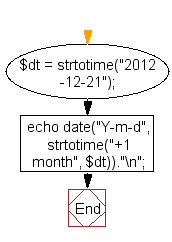﻿ PHP Date Exercise: Increment date by one month - w3resource# PHP Date Exercises : Increment date by one month

## PHP date: Exercise-24 with Solution

Write a PHP script to increment date by one month.

Sample date : 2012-12-21

Sample solition:

PHP Code:

``````<?php
\$dt = strtotime("2012-12-21");
echo date("Y-m-d", strtotime("+1 month", \$dt))."\n";
?>
```
```

Sample Output:

```2013-01-21
```

Flowchart :PHP Code Editor:

Have another way to solve this solution? Contribute your code (and comments) through Disqus.

What is the difficulty level of this exercise?

﻿

## PHP: Tips of the Day

define() vs. const

As of PHP 5.3 there are two ways to define constants: Either using the const keyword or using the define() function:

```const FOO = 'BAR';
define('FOO', 'BAR');
```

The fundamental difference between those two ways is that const defines constants at compile time, whereas define defines them at run time. This causes most of const's disadvantages. Some disadvantages of const are:

• const cannot be used to conditionally define constants. To define a global constant, it has to be used in the outermost scope:
```if (...) {
const FOO = 'BAR';    // Invalid
}
// but
if (...) {
define('FOO', 'BAR'); // Valid
}
```

Why would you want to do that anyway? One common application is to check whether the constant is already defined:

```if (!defined('FOO')) {
define('FOO', 'BAR');
}
```
• const accepts a static scalar (number, string or other constant like true, false, null, __FILE__), whereas define() takes any expression. Since PHP 5.6 constant expressions are allowed in const as well:
```const BIT_5 = 1 << 5;    // Valid since PHP 5.6 and invalid previously
define('BIT_5', 1 << 5); // Always valid
```
• const takes a plain constant name, whereas define() accepts any expression as name. This allows to do things like this:
```for (\$i = 0; \$i < 32; ++\$i) {
define('BIT_' . \$i, 1 << \$i);
}
```
• consts are always case sensitive, whereas define() allows you to define case insensitive constants by passing true as the third argument (Note: defining case-insensitive constants is deprecated as of PHP 7.3.0.):
```define('FOO', 'BAR', true);
echo FOO; // BAR
echo foo; // BAR
```
• So, that was the bad side of things. Now let's look at the reason why I personally always use const unless one of the above situations occurs:

• const simply reads nicer. It's a language construct instead of a function and also is consistent with how you define constants in classes.
• const, being a language construct, can be statically analysed by automated tooling.
• const defines a constant in the current namespace, while define() has to be passed the full namespace name:
```namespace A\B\C;
// To define the constant A\B\C\FOO:
const FOO = 'BAR';
define('A\B\C\FOO', 'BAR');```
• Since PHP 5.6 const constants can also be arrays, while define() does not support arrays yet. However, arrays will be supported for both cases in PHP 7.
```const FOO = [1, 2, 3];    // Valid in PHP 5.6
define('FOO', [1, 2, 3]); // Invalid in PHP 5.6 and valid in PHP 7.0```

Finally, note that const can also be used within a class or interface to define a class constant or interface constant. define cannot be used for this purpose:

```class Foo {
const BAR = 2; // Valid
}
// But
class Baz {
define('QUX', 2); // Invalid
}
```

Summary

Unless you need any type of conditional or expressional definition, use consts instead of define()s - simply for the sake of readability!

Ref : https://bit.ly/3h7VDPx